# Perimeter

The perimeter of a 2D shape, such as square, rectangle or triangle, is the sum of all the sides of the shape. For a circle, the perimeter is the length of the boundary of the circle called the circumference..

## Perimeter of Common Shapes

Let's look at how to calculate the perimeter of the shapes below:

### Triangle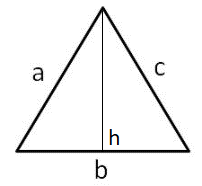The perimeter of a triangle is calculated by:

$$P = a + b + c$$

### Square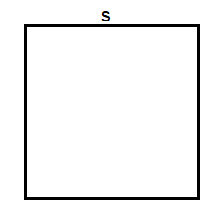The perimeter of a square with length s is calculated by:

$$P = 4s$$

### Rectangle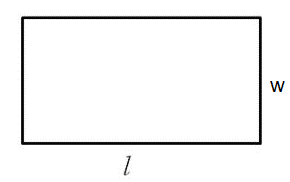The perimeter of a rectangle with sides l and h is calculated by:

$$P = 2*l + 2*w$$

### Circle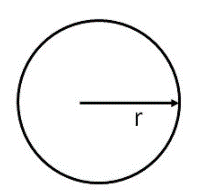The perimeter of a circle with radius r is calculated by:

$$P = 2 \pi r$$

### Trapezoid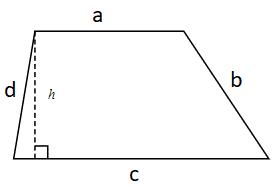The perimeter of a trapezoid with side lengths a, b, c and dis calculated by:

$$P = a + b + c + d$$

### Parallelogram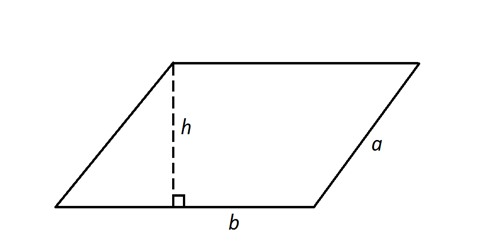The perimeter of a parallelogram with side lengthsl and w is calculated by:

$$P = 2 \cdot a + 2 \cdot b$$

An isosceles triangle has a perimeter of $$44$$ m. The base is $$10$$ m long. What is the length of the other sides?

Find the perimeter of the semi-circle below: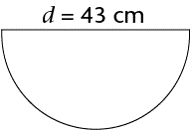An analog clock with a radius of 20 cm is being observed. The second hand, with its arrows touching the outer edge of the clock, spun 3 full rotations (3 min). What's the total distance the second hand's arrow travelled?